# HMs DIY #1-6

Store Link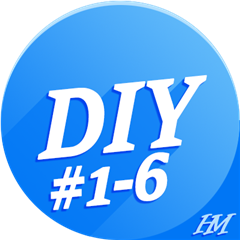Because I want to keep this datafield and the app free for use I would be very grateful about a small donation to PayPal

A detailed description of how to use it can be found here:

Thanks very much to mcinner1 for putting so much time into this documentation!

You can contribute on my github page.
You can upload your layout so others can use it as well.
Also any changes to the fonts.txt can be updated there as well.

Garmin_HMsDIY

=======================================================================
RELEASE NOTES
=======================================================================
-----------------------------------------------------------------------
3.4.1
* switched to new SDK 4.1.3
* added devices: Instinct 2S / Solar / Dual Power, D2 Air X10, D2 Mach 1, Descent G1 / G1 Solar,
Edge 1040 / 1040 Solar, Forerunner 255, Forerunner 255 Music, Forerunner 255s, Forerunner 255s Music, Forerunner 955 / Solar, Instinct 2 / Solar / Dual Power / dezl Edition
* fixed crash when windspeed was not available from device
-----------------------------------------------------------------------
3.3.1
* switched to new SDK 4.0.9
* added devices: D2 Air, Descent MK2/MK2i, Descent Mk2S, Enduro, epix Gen2, Fenix 7, Fenix 7S, Fenix 7X, Forerunner 55, Forerunner 945 LTE, Venu2, Venu2 Plus,
Venus 2S, Venu Sq, Venu Sq Music,
-----------------------------------------------------------------------
3.2.1
* fixed a bug in an array when calculating average of many values
* datafield is now shown to support all languages available (because everyone can enter his own language of course)
* switched to new SDK 4.0.1
* new values: Total season ascent (similar to odometer, value will be stored in the settings until manually reset), Current lap ascent

for full releasenotes see: releasenotes.txt
=======================================================================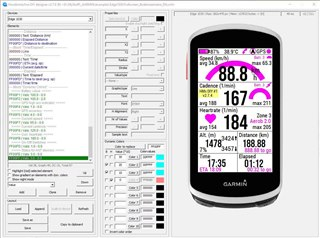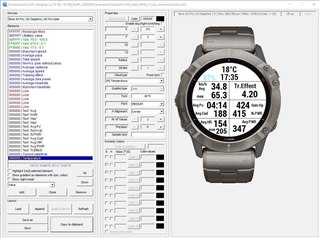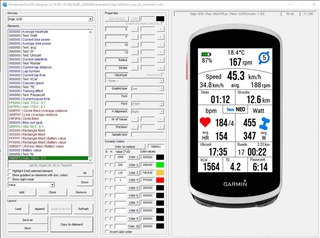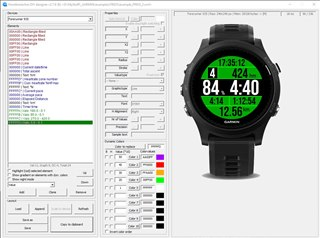• I am very pleased that you dare to follow in the footsteps of easuvorov, whose DIY I greatly appreciate. Unfortunately, he retired and I hoped that someone would come up with this great idea.
I will start testing tomorrow with great interest.
All the best!

• Hi

Thank you...

Please be aware that no fonts are adjusted yet, except for the FR935. I had no time to do it yet.

If the basic stuff works, I will add more values and features...

Regards

Erich

• Hello

I am looking forward to playing with it.

Initial feedback:

• I think it would be nice if the preview would be in another colour than white since white text is difficult to see as highlighted.
• Also, maybe add new elements in the middle so it's easier to see in the builder they have been added?
• Holdning down "+" should continuously move an element
• When I select battery value the value disappears (see attached XML)

Questions:

• What does Nr. of Values do?
• Can I make a lap value (avg last lap HR for example)?
• Can I decide number of decimals/seconds?

```<?xml version="1.0" encoding="UTF-8"?>
<DIYLayout>
<Device name="Forerunner® 935"/>
<LayoutElement type="1" color="0000FF" x="7" y="153" x2="233" y2="238" eletype="RF" radius="0" stroke="1"/>
<LayoutElement type="1" color="00AAFF" x="120" y="120" x2="0" y2="0" eletype="CR" radius="119" stroke="3"/>
<LayoutElement type="1" color="00AAFF" x="9" y="80" x2="233" y2="80" eletype="LN" radius="0" stroke="2"/>
<LayoutElement type="1" color="00AAFF" x="6" y="153" x2="235" y2="153" eletype="LN" radius="0" stroke="2"/>
<LayoutElement type="1" color="00AAFF" x="112" y="153" x2="112" y2="189" eletype="LN" radius="0" stroke="2"/>
<LayoutElement type="1" color="00AAFF" x="23" y="189" x2="216" y2="189" eletype="LN" radius="0" stroke="2"/>
<LayoutElement type="0" color="555555" x="165" y="14" eletype="FX" text="%" font="XTINY" halignment="1" precision="0" nr_values="1"/>
<LayoutElement type="0" color="AAAAAA" x="188" y="163" eletype="FX" text="km" font="XTINY" halignment="2" precision="0" nr_values="1"/>
<LayoutElement type="0" color="FFFFFB" x="119" y="15" eletype="HP" text="" font="NR_THAI_HOT" halignment="1" precision="0" nr_values="1"/>
<LayoutElement type="0" color="FFFFFA" x="25" y="56" eletype="HR" text="" font="XTINY" halignment="2" precision="0" nr_values="1"/>
<LayoutElement type="0" color="FFFFFC" x="120" y="86" eletype="CP" text="" font="NR_THAI_HOT" halignment="1" precision="0" nr_values="5"/>
<LayoutElement type="0" color="AAAAAA" x="68" y="156" eletype="AP" text="" font="NR_MILD" halignment="1" precision="0" nr_values="1"/>
<LayoutElement type="0" color="AAAAAA" x="187" y="156" eletype="DS" text="" font="NR_MILD" halignment="0" precision="2" nr_values="1"/>
<LayoutElement type="0" color="AAAAAA" x="120" y="194" eletype="TT" text="" font="NR_MILD" halignment="1" precision="0" nr_values="1"/>
<LayoutElement type="2" replDC="FFFFFA" valDC00="1860" colDC00="AA00FF" valDC01="1680" colDC01="FF0000" valDC02="1500" colDC02="FFAA00" valDC03="1370" colDC03="00FF00" valDC04="1" colDC04="FFFFFF" invertcolor="false"/>
<LayoutElement type="2" replDC="FFFFFB" valDC00="950" colDC00="AA00FF" valDC01="860" colDC01="FF0000" valDC02="760" colDC02="FFAA00" valDC03="700" colDC03="00FF00" valDC04="1" colDC04="FFFFFF" invertcolor="false"/>
<LayoutElement type="2" replDC="FFFFFC" valDC00="45" colDC00="800080" valDC01="50" colDC01="FF0000" valDC02="55" colDC02="FF5500" valDC03="60" colDC03="00FF00" valDC04="65" colDC04="0055FF" valDC05="70" colDC05="FFFFFF" invertcolor="true"/>
</DIYLayout>
```

• Hi

Thanks for taking a look at it!

Battery value should be shown in next release, forgot the "default" text in the designer.

I will change the highlight color to red.

Regarding the +/- buttons: I will try to add a handler for continous pressing, but it should already work with

the enter key to auto increment/decrement.

A new element is added just below the current selection, so you can control it yourself where it is in the list. You

can also move it up/down later.

Nr of values averages the value over time. Because the values are updated every second, nr of values is the averaged

value over XX seconds.

I didn't add lap value yet, but it will be in the next release.

You can set the number of decimals with precision. Time and pace strings are in fixed format for now. (XXmin:XXsec)

regards

Erich

• Thank you for the reply.

I tried installing and adding a code to my Fenix 5+, latest firmware.

When I add it as a data screen I get the IQ! error. I think it even crashed the Run application and I had to restart it.

```<?xml version="1.0" encoding="UTF-8"?>
<DIYLayout>
<Device name="fenix® 5S Plus"/>
<LayoutElement type="1" color="FFFFFF" x="120" y="120" x2="0" y2="0" eletype="CF" radius="120" stroke="1"/>
<LayoutElement type="0" color="000000" x="120" y="12" eletype="TT" text="" font="S_NR_MILD" halignment="1" precision="0" nr_values="0"/>
<LayoutElement type="1" color="0000FF" x="0" y="50" x2="240" y2="50" eletype="LN" radius="0" stroke="3"/>
<LayoutElement type="0" color="000000" x="13" y="55" eletype="DS" text="" font="NR_MEDIUM" halignment="2" precision="0" nr_values="2"/>
<LayoutElement type="1" color="8000FF" x="0" y="100" x2="240" y2="100" eletype="LN" radius="0" stroke="3"/>
<LayoutElement type="0" color="000000" x="0" y="110" eletype="CP" text="" font="NR_HOT" halignment="2" precision="0" nr_values="2"/>
<LayoutElement type="0" color="C0C0C0" x="236" y="107" eletype="AP" text="" font="NR_MEDIUM" halignment="0" precision="0" nr_values="2"/>
<LayoutElement type="1" color="C0C0C0" x="0" y="170" x2="240" y2="170" eletype="LN" radius="0" stroke="3"/>
<LayoutElement type="0" color="FF80C0" x="50" y="180" eletype="HR" text="" font="NR_MEDIUM" halignment="2" precision="0" nr_values="2"/>
<LayoutElement type="2" replDC="FF80C0" valDC00="160" colDC00="FF0000" valDC01="147" colDC01="FF8000" valDC02="139" colDC02="00FF40" valDC03="127" colDC03="0080FF" invertcolor="false"/>
<LayoutElement type="0" color="C0C0C0" x="198" y="173" eletype="CA" text="" font="MEDIUM" halignment="0" precision="0" nr_values="2"/>
</DIYLayout>
```

• I had to uninstall it as it crashed my Run activity.

• Hi

Sorry for that. I have found the problem. The nr of values must not be zero. Unfortunately I did not add a check in the designer, so if you set the nr_of_values of timer time to 1 it should work.

I will add a check in the next version.

One more thing I noticed. Values for dynamic colors have to be multiplied by 10 (to get 1/10th precision)!

regards

Erich

• New version of the designer is out.

Releasenotes:

1.1.1
* fixed empty battery value in designer
* added a check for nr_of_values to be greater than zero
* selected element is now red

1.1.0 initial release

• Your designer tool works well and intuitively.

The first thing I did was to compare the fonts of the designer and the actual Edge device. That also looks good!
For texts use XTINY up to LARGE and for numbers better use the NR_MILD to NR_HOT fonts.

So far my testings now.

I'm happy to do any tests you can think of on the Edge 1000 and 1030 for you.